# RD Sharma Class 8 Solutions Chapter 6 Algebraic Expressions and Identities Ex 6.2

In this chapter, we provide RD Sharma Class 8 Solutions Chapter 6 Algebraic Expressions and Identities Ex 6.2 for English medium students, Which will very helpful for every student in their exams. Students can download the latest RD Sharma Class 8 Solutions Chapter 6 Algebraic Expressions and Identities Ex 6.2 Maths pdf, free RD Sharma Class 8 Solutions Chapter 6 Algebraic Expressions and Identities Ex 6.2 Maths book pdf download. Now you will get step by step solution to each question.

### RD Sharma Solutions for Class 8 Chapter 6 Algebraic Expressions and Identities Ex 6.2Download PDF

Question 1.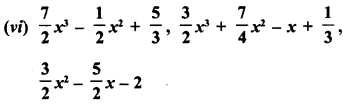Solution: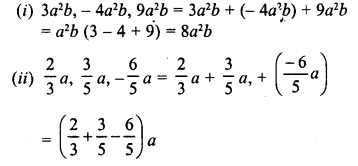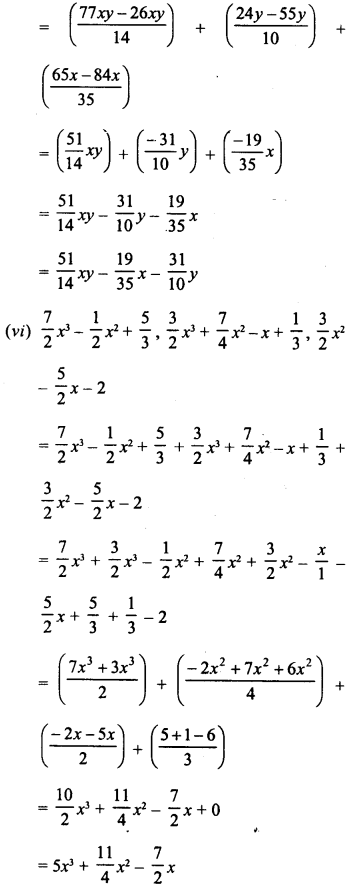Question 2.
Subtract:
(i) -5xy from 12xy
(ii) 2a2 from -7a2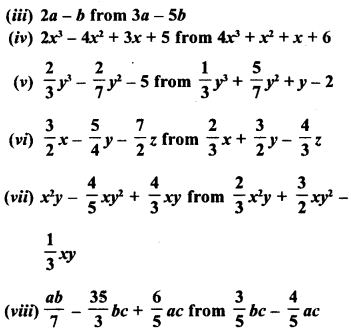Solution: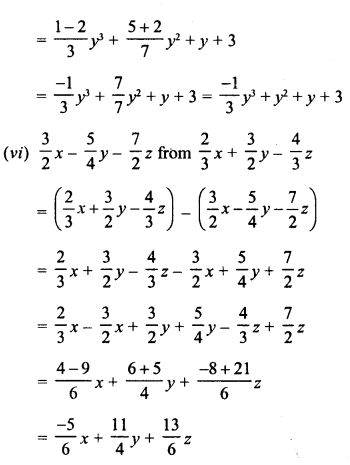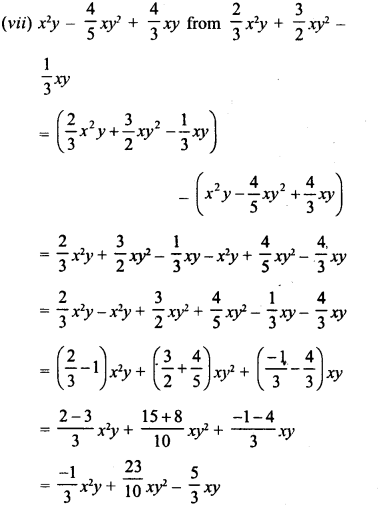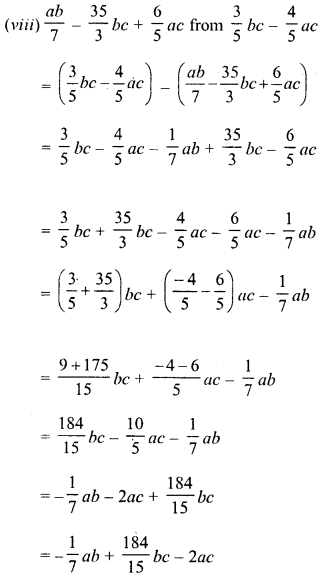Question 3.
Take away :Solution: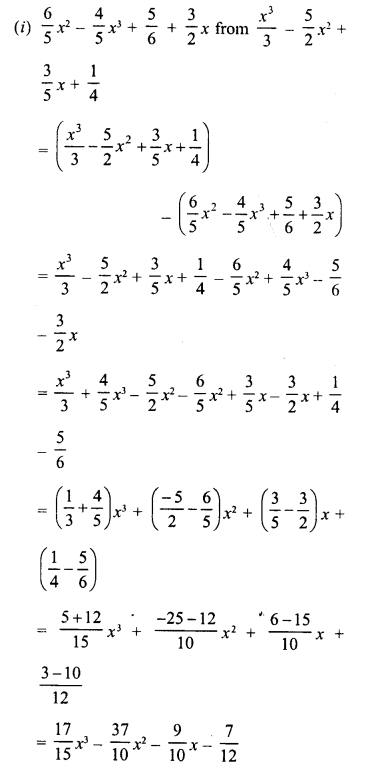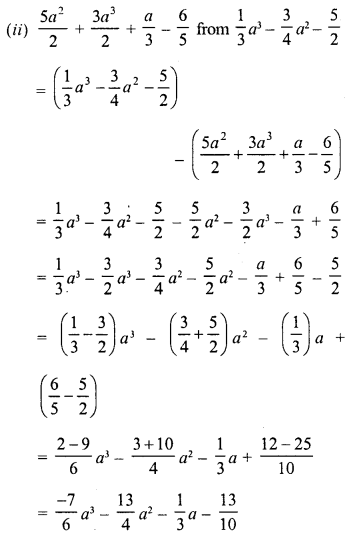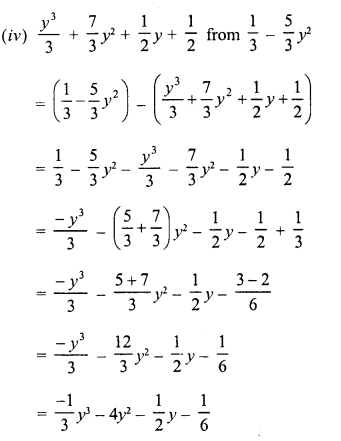Question 4.
Subtract 3x – 4y – 7z from the sum of x – 3y + 2z and – 4X + 9y- 11z.
Solution:
Sum of x – 3y + 2z and – 4x + 9y – 11z
= x – 3y + 2z + (- 4x + 9y – 11z)
= x – 3y + 2z – 4x + 9y – 11z
= x – 4x – 3y + 9y + 2z – 11z
= – 3x + 6y – 9z
Now (-3x + 6y – 9z) – (3x – 4y – 7z)
= -3x + 6y – 9z – 3x + 4y + 7z
= -3x – 3x + 6y + 4y -9z +7z
= -6x + 10y – 2z

Question 5.
Subtract the sum of 3l- 4m – 7n2 and 2l + 3m – 4n2 from the sum of 9l + 2m – 3nand -3l + m + 4n2.

Solution:
Sum of 9l + 2m – 3n2 and -3l + m + 4n2
= 9l + 2m – 3 n2 + (-3l) + m + 4n2
= 9l + 2m – 3n2 – 3l + m + 4n2
= 9l- 3l+ 2m + m – 3 n2 + 4n2
= 6l + 3m + n2
and sum of 3l – 4m – 7n2 and 2l +3m- 4n2
= 3l- 4m – 7n2 + 2l+ 3m- 4n2
= 3l + 2l – 4m + 3m- 7n2 – 4n2
= 5l -m- 11n2
Now (6l + 3m + n2) – (5l – m – 11n2)
= 6l + 3m + n2 – 5l + m + 11n2
= 6l – 5l + 3m + m + n2 + 11n2
= l + 4m+ 12n2

Question 6.
Subtract the sum of 2x – x2 + 5 and -4x – 3 + 7x2 from 5.
Solution:
5 – (2x-x2 + 5-4x-3 + 7x2)
= 5 – (2x – 4x- x2 + 7x2 + 5-3)
= 5 – (-2x + 6x2 + 2)
= 5 + 2x – 6x2 – 2
= – 6x2+2x+3
= 3 + 2x – 6x2

Question 7.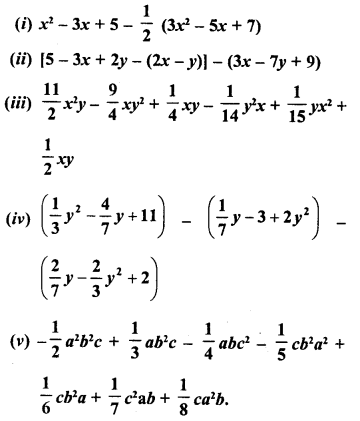Solution: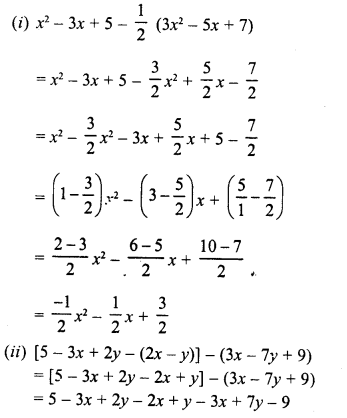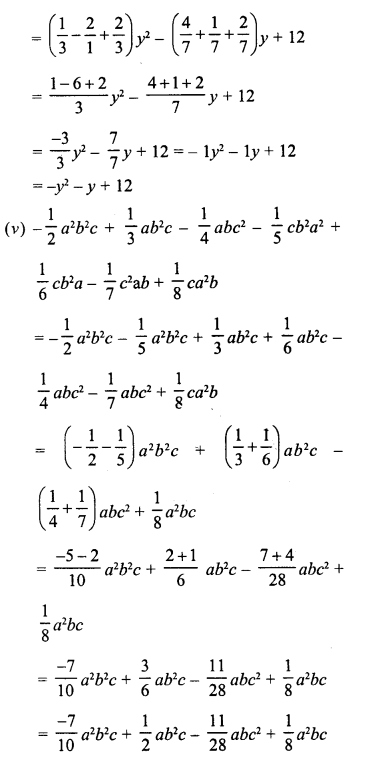All Chapter RD Sharma Solutions For Class 8 Maths

—————————————————————————–

All Subject NCERT Exemplar Problems Solutions For Class 8

All Subject NCERT Solutions For Class 8

*************************************************

I think you got complete solutions for this chapter. If You have any queries regarding this chapter, please comment on the below section our subject teacher will answer you. We tried our best to give complete solutions so you got good marks in your exam.

If these solutions have helped you, you can also share rdsharmasolutions.in to your friends.